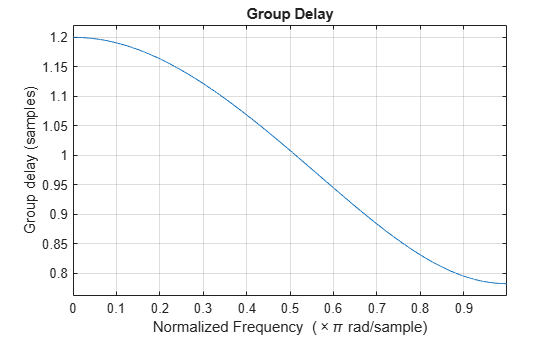Documentation

### This is machine translation

Mouseover text to see original. Click the button below to return to the English version of the page.

Note: This page has been translated by MathWorks. Click here to see
To view all translated materials including this page, select Country from the country navigator on the bottom of this page.

# fdesign.fracdelay

Fractional delay filter specification object

## Syntax

```d = fdesign.fracdelay(delta) d = fdesign.fracdelay(delta,'N') d = fdesign.fracdelay(delta,'N',n) d = fdesign.fracdelay(delta,n) d = fdesign.fracdelay(...,fs) ```

## Description

`d = fdesign.fracdelay(delta)` constructs a default fractional delay filter designer `d` with the filter order set to 3 and the delay value set to `delta`. The fractional delay `delta` must be between 0 and 1 samples.

`d = fdesign.fracdelay(delta,'N')` initializes the filter designer specification to `N`, where `N` specifies the fractional delay filter order and defaults to filter order of 3.

Use `designmethods``(d)` to get a list of the design methods available for a specification.

`d = fdesign.fracdelay(delta,'N',n)` initializes the filter designer to `N` and sets the filter order to `n`.

`d = fdesign.fracdelay(delta,n)` assumes the default specification `N`, filter order, and sets the filter order to the value you provide in input `n`.

`d = fdesign.fracdelay(...,fs)` adds the argument `fs`, specified in units of Hertz (Hz) to define the sampling frequency. In this case, specify the fractional delay `delta` to be between 0 and 1/`fs`.

## Examples

collapse all

Design a second-order fractional delay filter of 0.2 samples using the Lagrange method. Implement the filter using a Farrow fractional delay (fd) structure.

```d = fdesign.fracdelay(0.2,'N',2); secondOrderFrac = design(d,'lagrange','filterstructure','farrowfd'); fvtool(secondOrderFrac,'analysis','grpdelay')```Design a cubic fractional delay filter with a sampling frequency of 8 kHz and fractional delay of 50 microseconds using the Lagrange method.

```d = fdesign.fracdelay(50e-6,'N',3,8000); cubicFrac = design(d, 'lagrange', 'FilterStructure', 'farrowfd');```

Watch now